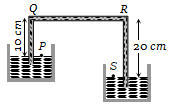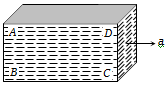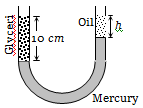A siphon in use is demonstrated in the following figure. The density of the liquid flowing in siphon is 1.5 gm/cc. The pressure difference between the point P and S will be(a)                                  (b)

(c) Zero                                        (d) Infinity

Concept Questions :-

Pressure
High Yielding Test Series + Question Bank - NEET 2020

Difficulty Level:

The height of a mercury barometer is 75 cm at sea level and 50 cm at the top of a hill. Ratio of density of mercury to that of air is ${10}^{4}$. The height of the hill is
(a) 250 m                              (b) 2.5 km
(c) 1.25 km                            (d) 750 m

Concept Questions :-

Pressure
High Yielding Test Series + Question Bank - NEET 2020

Difficulty Level:

Equal masses of water and a liquid of relative density 2 are mixed together, then the mixture has a density of
(a) 2/3                                 (b) 4/3
(c) 3/2                                  (d) 3

High Yielding Test Series + Question Bank - NEET 2020

Difficulty Level:

A body of density ${\mathrm{d}}_{1}$ is counterpoised by Mg of weights of density ${\mathrm{d}}_{2}$ in air of density d. Then the true mass of the body is

(a) M                                    (b) $\mathrm{M}\left(1-\frac{\mathrm{d}}{{\mathrm{d}}_{2}}\right)$

(c) $\mathrm{M}\left(1-\frac{\mathrm{d}}{{\mathrm{d}}_{1}}\right)$                       (d) $\frac{\mathrm{M}\left(1-\mathrm{d}/{\mathrm{d}}_{2}\right)}{\left(1-\mathrm{d}/{\mathrm{d}}_{1}\right)}$

Concept Questions :-

Archimedes principle
High Yielding Test Series + Question Bank - NEET 2020

Difficulty Level:

The value of g at a place decreases by 2%. The barometric height of mercury
(a) Increases by 2%
(b) Decreases by 2%
(c) Remains unchanged
(d) Sometimes increases and sometimes decreases

Concept Questions :-

Pressure
High Yielding Test Series + Question Bank - NEET 2020

Difficulty Level:

A barometer kept in a stationary elevator reads 76 cm. If the elevator starts accelerating up, the reading will be
(a) Zero                                       (b) Equal to 76 cm
(c) More than 76 cm                     (d) Less than 76 cm

Concept Questions :-

Pressure
High Yielding Test Series + Question Bank - NEET 2020

Difficulty Level:

A closed rectangular tank is completely filled with water and is accelerated horizontally with an acceleration a towards right. Pressure is (i) maximum at, and (ii) minimum at(a) (i) B (ii) D
(b) (i) C (ii) D
(c) (i) B (ii) C
(d) (i) B (ii) A

Concept Questions :-

Pressure
High Yielding Test Series + Question Bank - NEET 2020

Difficulty Level:

A beaker containing a liquid is kept inside a big closed jar. If the air inside the jar is continuously pumped out, the pressure in the liquid near the bottom of the beaker will
1. Increase
2. Decrease
3. Remain constant
4. First decrease and then increase

Concept Questions :-

Pressure
High Yielding Test Series + Question Bank - NEET 2020

Difficulty Level:

A barometer tube reads 76 cm of mercury. If the tube is gradually inclined at an angle of 60o with vertical, keeping the open end immersed in the mercury reservoir, the length of the mercury column will be
(a) 152 cm                               (b) 76 cm
(c) 38 cm                                 (d) $38\sqrt{3}$ cm

Concept Questions :-

Pressure
High Yielding Test Series + Question Bank - NEET 2020

Difficulty Level:

A vertical U-tube of uniform inner cross section contains mercury in both sides of its arms. A glycerin (density = 1.3 g/${\mathrm{cm}}^{3}$) column of length 10 cm is introduced into one of its arms. Oil of density 0.8 gm/${\mathrm{cm}}^{3}$ is poured into the other arm until the upper surfaces of the oil and glycerin are in the same horizontal level. Find the length of the oil column. [Density of mercury = 13.6 g/${\mathrm{cm}}^{3}$](a) 10.4 cm               (b) 8.2 cm

(c) 7.2 cm                  (d) 9.6 cm

Concept Questions :-

Pressure